# Poisson distribution - daisies

The meadow behind FLD was divided into 100 equally large parts. Subsequently, it was found that there were no daisies in ten of these parts. Estimate the total number of daisies in the meadow. Assume that daisies are randomly distributed in the meadow.

n =  230

### Step-by-step explanation: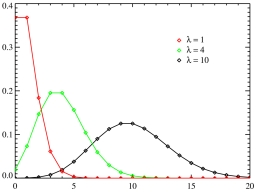Did you find an error or inaccuracy? Feel free to write us. Thank you!Tips to related online calculators
Looking for a statistical calculator?
Do you have a linear equation or system of equations and looking for its solution? Or do you have a quadratic equation?
Do you want to round the number?
Would you like to compute count of combinations?

#### You need to know the following knowledge to solve this word math problem:

We encourage you to watch this tutorial video on this math problem:

## Related math problems and questions:

• SeedlingsThe meadow was divided into 30 equally large parts and then it was calculated how many seedlings are in each part. It was found that there were no seedlings in six parts, five parts contained one seedling, twelve parts two seedlings and seven parts three
• Family94 boys are born per 100 girls. Determine the probability that there are two boys in a randomly selected family with three children.
• Twenty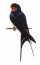Twenty swallows sit on a 10 m long telephone cable. Assume that swallows are completely randomly distributed along the line. (a) What is the probability that more than three swallows sit on a randomly selected section of cable 1 m long? (b) What is the pr
• SeedsFrom a box of spruce seeds with the germination of 80%, we randomly select 10 seeds and plant them. Find the median of the random variable: the number of germinating seeds.
• DeficienciesDuring the hygienic inspection in 2000 mass caterers, deficiencies were found in 300 establishments. What is the probability that deficiencies in a maximum of 3 devices will be found during the inspection of 10 devices?
• TV failThe TV has after 10,000 hours average 35 failures. Determine the probability of TV failure after 400 hours of operation.
• RecordsRecords indicate 90% error-free. If 8 records are randomly selected, what is the probability that at least 2 records have no errors?
• Bernoulli distribution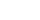The production of solar cells produces 2% of defective cells. Assume the cells are independent and that a lot contains 800 cells. Approximate the probability that less than 20 cells are defective. (Answer to the nearest 3 decimals).
• Probability of failures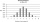In certain productions, the probability of failures is 0.01. Calculate the probability that there will be more than one failure among the 100 selected products if we return the selected products to the file after the check.
• Population variance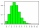In a California community college, 60% of students will transfer to a college in the CSU system. The number of students in a sample who will transfer follows a binomial distribution. If 8 students are randomly selected, find the population variance σ2 of
• Exponential equationFind x, if 625 ^ x = 5 The equation is exponential because the unknown is in the exponential power of 625
• Binomial probability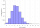What is the binominal probability that at least 4 of the 6 trials (n=6) are succeeded where φ = 0.50?
• DiceWe throw five times the dice. What is the probability that six fits exactly twice?
• FamilyWhat is the probability that a family with 7 childrens have: exactly 6 dívek? 2 dívky and 5 boys? Consider the birth probability of a girl is 48.87% and boy 51.13%.
• Sample ProportionIn a California community college, 60% of students will transfer to a college in the CSU system. The number of students in a sample who will transfer follows a binomial distribution. 400 students are randomly selected from the college and 224 of them will
• SalesFrom statistics of sales goods, item A buy 51% of people and item B buys 59% of people. What is the probability that from 10 people buy 2 item A and 8 item B?
• Families 2Seven hundred twenty-nine families are having six children each. The probability of a girl is 1/3, and the probability of a boy is 2/3. Find the number of families having two girls and four boys.